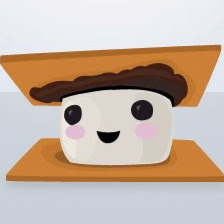# Bluetooth Controlled Thermostat

I created a device that can be mounted onto the dormitory thermostats on John Brown University's campus. It is controlled by a mobile app.

IntermediateShowcase (no instructions)1 hour435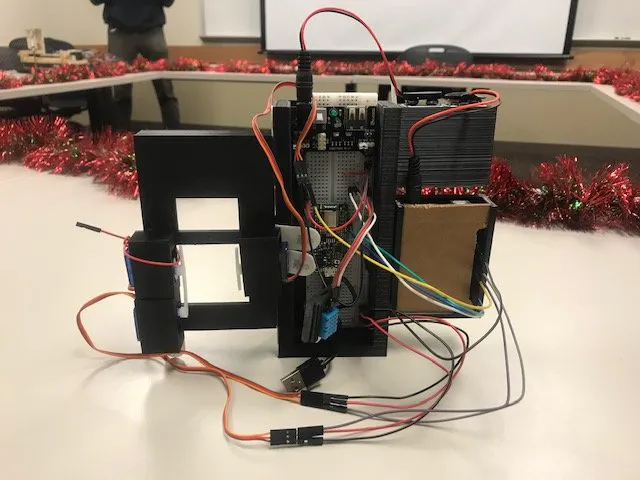## Things used in this project

### Hardware componentsArduino UNO
×1
×1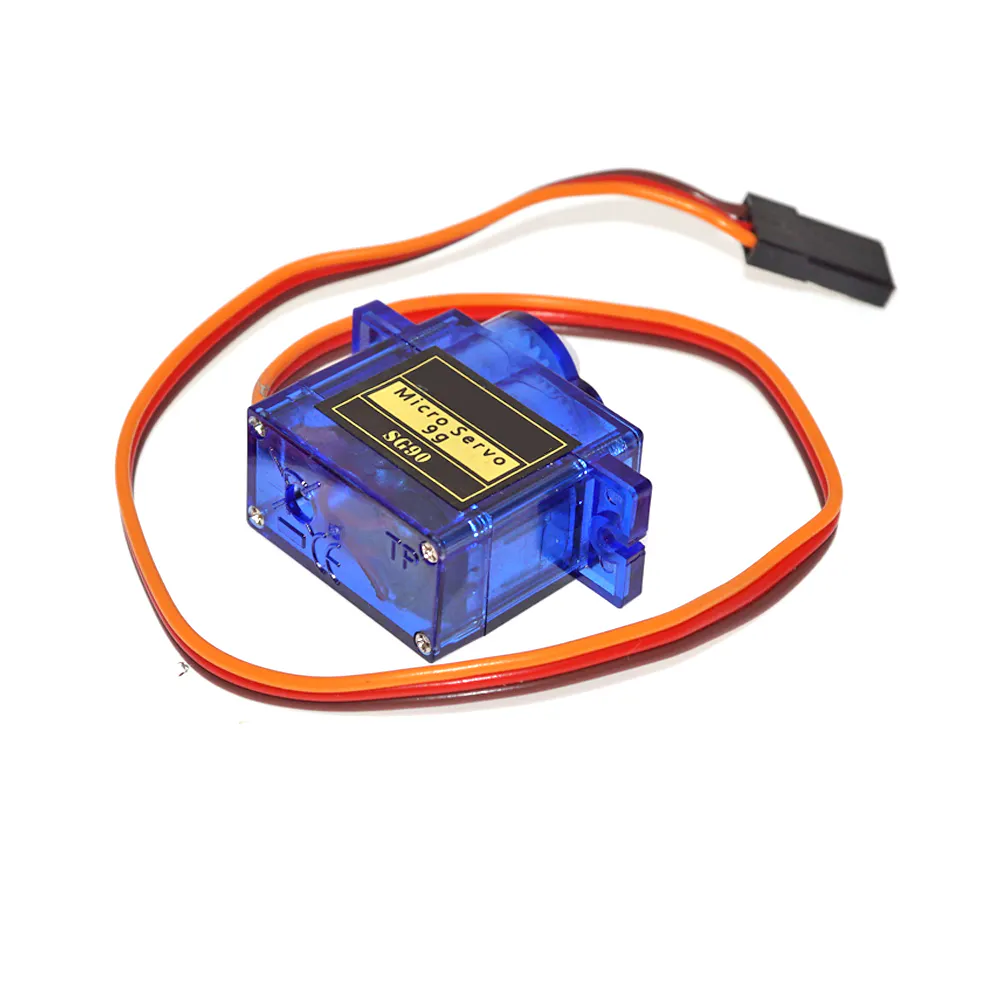SG90 Micro-servo motor
×3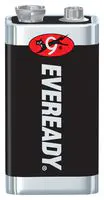Battery, 9 V
×2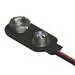9V Battery Clip
×2
×1

### Hand tools and fabrication machinesSoldering iron (generic)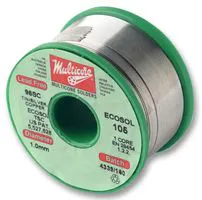3D Printer (generic)

## Custom parts and enclosures

### Wall Mount for Project

This is the 3D modeled wall mount for the project. Created in Solidworks

## Schematics

### Circuit Diagram

Follow the wires and connect them accordingly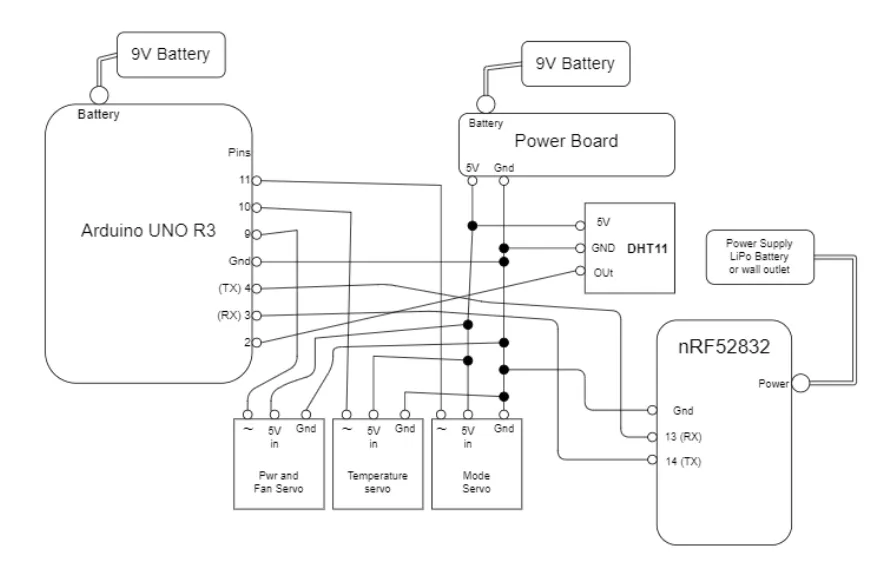## Code

Arduino
Make sure the appropriate libraries are included. Going to Adafruit's tutorial for the nRF52832 will provide you with what you need
```#include <SoftwareSerial.h>
#include <InternalFileSystem.h>
#include <bluefruit.h>

// OTA DFU service
BLEDfu  bledfu;
BLEBas  blebas;  // battery
BLEDis  bledis;  // device information
// Uart over BLE service
BLEUart bleuart;

// Function prototypes for packetparser.cpp
uint8_t readPacket (BLEUart *ble_uart, uint16_t timeout);
float   parsefloat (uint8_t *buffer);
void    printHex   (const uint8_t * data, const uint32_t numBytes);

// Packet buffer
extern uint8_t packetbuffer[];
int condIn = A5;
int condition = 0;

//Serial Communication to Arduino
SoftwareSerial Arduino(13, 14); //RX, TX
String inString = " "; //string to hold communication input
int Flag = 0;
int Input = 0;

//Settings Responses
int pflag = 0;

int SpeedIndex = 1;

int Ctemperature = 67;
int Htemperature = 40;
int DryTemp = 67;
int AutoTempH = 55;
int AutoTempC = 75;
int BackTempH = 55;
int BackTempC = 75;
int SetFlag = 0;
int TempFlag = 0;
int Press = 0;
int Press2 = 0;
int Press3 = 0;
int Press4 = 0;
int Press5 = 0;
int SetC = 0;
int SetH = 0;
int ModeIndex = 0;

//integer to ASCII variables
int temp = 0;
int tens = 0;
int ones = 0;
int Temp10 = 0;
int Temp1 = 0;
char bluff[] = {'0','1','2','3','4','5','6','7','8','9'}; //indexing starts at 0;

void setup(void)
{
pinMode(condIn, INPUT);
Serial.begin(115200);
while ( !Serial ) delay(10);   // for nrf52840 with native usb

Bluefruit.begin();
Bluefruit.setTxPower(4);    // Check bluefruit.h for supported values
Bluefruit.setName("AC Controller");

// Configure and Start Device Information Service
bledis.setManufacturer("Josiah Heikes");
bledis.setModel("AC Controller V1.0");
bledis.begin();
// To be consistent OTA DFU should be added first if it exists
bledfu.begin();

// Start BLE Battery Service
blebas.begin();
blebas.write(100);

// Configure and start the BLE Uart service
bleuart.begin();

// Set up and start advertising

// Serial.println(F("Please use Adafruit Bluefruit LE app to connect in Controller mode"));
//  Serial.println(F("Then activate/use the sensors, color picker, game controller, etc!"));
//  Serial.println();
Arduino.begin(115200);
Arduino.println("This is just the beginning");
}

{

// Include the BLE UART (AKA 'NUS') 128-bit UUID

// Secondary Scan Response packet (optional)
// Since there is no room for 'Name' in Advertising packet

* - Enable auto advertising if disconnected
* - Interval:  fast mode = 20 ms, slow mode = 152.5 ms
* - Timeout for fast mode is 30 seconds
* - Start(timeout) with timeout = 0 will advertise forever (until connected)
*
* https://developer.apple.com/library/content/qa/qa1931/_index.html
*/
Bluefruit.Advertising.setInterval(32, 244);    // in unit of 0.625 ms
Bluefruit.Advertising.setFastTimeout(30);      // number of seconds in fast mode
}

void loop(void)
{
Arduino.listen();
if (len == 0) return;

// Got a packet!
// printHex(packetbuffer, len);

// Buttons
if (packetbuffer == 'B') {
uint8_t buttnum = packetbuffer - '0';
boolean pressed = packetbuffer - '0';
// Serial.print ("Button "); Serial.print(buttnum);
if((buttnum == 1)&&pressed) {
Arduino.write(1);
power();
}
if((buttnum == 2)&&pressed) {
Arduino.write(2);
fan();
}
if((buttnum == 3)&&pressed){
Arduino.write(3);
CurrentMode();
}
if((buttnum == 4)&&pressed){
Arduino.write(4);
}
if((buttnum == 5)&&pressed){
Arduino.write(5);
TempUP();
}
if((buttnum == 6)&&pressed){
Arduino.write(6);
TempDown();
}
if((buttnum == 8)&&pressed){
Arduino.write(8);
MODE();
}
}
}

void ToASCII(int Val)
{
ones = Val%10;
tens = (Val-ones)/10;
}
void power(){
if(pflag == 0){
pflag = 1;
bleuart.write("\rPower On");
}else{
pflag = 0;
bleuart.write("\rPower Off");
}
}

void fan(){
if(pflag == 1){
SpeedIndex += 1;
if(SpeedIndex > 4){
SpeedIndex = 1;
}
bleuart.write("\rFan Speed: ");
bleuart.write(bluff[SpeedIndex]);
}else{
bleuart.write("\rTurn Power On");
}
}

void CurrentMode(){
bleuart.write("\rCurrent Mode: ");
if(ModeIndex == 0){
bleuart.write("Cool");
}else if(ModeIndex == 1){
bleuart.write("Drying");
}else if(ModeIndex == 2){
bleuart.write("Fan");
}else if(ModeIndex == 3){
bleuart.write("Auto");
}else if(ModeIndex == 4){
bleuart.write("Set-Back");
}else{
bleuart.write("Heat");
}
if(pflag == 0){
bleuart.write("\rPower Currently OFF");
}
}

void TempUP(){
if(pflag == 1){
if(ModeIndex == 0){
CoolingUP(Ctemperature);
Ctemperature = temp;
}
if(ModeIndex == 1){
DryTemp += 1;
if(DryTemp > 95){
DryTemp = 95;
bleuart.write("\rMax Drying Temp Reached");
}else{
bleuart.write("\rDry Temp Set to: ");
ToASCII(DryTemp);
bleuart.write(bluff[tens]);bleuart.write(bluff[ones]);
}
}
if(ModeIndex == 2){
bleuart.write("\rInvalid input");
}
if(ModeIndex == 3){
Press2 += 1;
if((Press2 == 1)&&(Press4 == 0)){
bleuart.write("\rAuto Temps(C/H): ");
FractionDisplay(AutoTempC, AutoTempH);
SetFlag = 1;
}if(((Press2 > 1)||(Press4 >= 1)) && (TempFlag == 0)){
CoolingUP(AutoTempC);
AutoTempC = temp;
HeatingUP(AutoTempH);
AutoTempH = temp;
bleuart.write("\rAuto Temps(C/H): ");
FractionDisplay(AutoTempC,AutoTempH);
SetFlag = 1;
}
if(SetC == 1){
CoolingUP(AutoTempC);
AutoTempC = temp;
bleuart.write("\rAuto Temps(C/H): ");
FractionDisplay(AutoTempC, AutoTempH);
}
if(SetH == 1){
HeatingUP(AutoTempH);
AutoTempH = temp;
bleuart.write("\rAuto Temps(C/H): ");
FractionDisplay(AutoTempC, AutoTempH);
}
}
if(ModeIndex == 4){
Press3 += 1;
if((Press3 == 1)&&(Press5 == 0)){
bleuart.write("\rSet-Back Temps(C/H): ");
FractionDisplay(BackTempC, BackTempH);
SetFlag = 1;
}else if(((Press3 > 1)||(Press5 >= 1)) && (TempFlag == 0)){
CoolingUP(BackTempC);
BackTempC = temp;
HeatingUP(BackTempH);
BackTempH = temp;
bleuart.write("\rSet-Back Temps(C/H): ");
FractionDisplay(BackTempC,BackTempH);
SetFlag = 1;
}
if(SetC == 1){
CoolingUP(BackTempC);
BackTempC = temp;
bleuart.write("\rSet-Back Temps(C/H): ");
FractionDisplay(BackTempC, BackTempH);
}
if(SetH == 1){
HeatingUP(BackTempH);
BackTempH = temp;
bleuart.write("\rSet-Back Temps(C/H): ");
FractionDisplay(BackTempC, BackTempH);
}
}
if(ModeIndex == 5){
HeatingUP(Htemperature);
Htemperature = temp;
}
}else{
bleuart.write("\rTurn Power On");
}
}

void TempDown(){
if(pflag == 1){
if(ModeIndex == 0){
CoolingDown(Ctemperature);
Ctemperature = temp;
}
if(ModeIndex == 1){
DryTemp -= 1;
if(DryTemp < 67){
DryTemp = 67;
bleuart.write("\rMin Drying Temp Reached");
}else{
bleuart.write("\rDry Temp Set to: ");
ToASCII(DryTemp);
bleuart.write(bluff[tens]);bleuart.write(bluff[ones]);
}
}
if(ModeIndex == 2){
bleuart.write("\rInvalid input");
}
if(ModeIndex == 3){
Press4 += 1;
if((Press4 == 1) && (Press2 == 0)){
bleuart.write("\rAuto Temps(C/H): ");
FractionDisplay(AutoTempC, AutoTempH);
SetFlag = 1;
}else if(((Press4 > 1)||(Press2 >= 1)) && (TempFlag == 0)){
CoolingDown(AutoTempC);
AutoTempC = temp;
HeatingDown(AutoTempH);
AutoTempH = temp;
bleuart.write("\rAuto Temps(C/H): ");
FractionDisplay(AutoTempC,AutoTempH);
SetFlag = 1;
}
if(SetC == 1){
CoolingDown(AutoTempC);
AutoTempC = temp;
bleuart.write("\rAuto Temps(C/H): ");
FractionDisplay(AutoTempC, AutoTempH);
}
if(SetH == 1){
HeatingDown(AutoTempH);
AutoTempH = temp;
bleuart.write("\rAuto Temps(C/H): ");
FractionDisplay(AutoTempC, AutoTempH);
}
}
if(ModeIndex == 4){
Press5 += 1;
if((Press5 == 1)&&(Press3 == 1)){
bleuart.write("\rSet-Back Temps(C/H): ");
FractionDisplay(BackTempC, BackTempH);
SetFlag = 1;
}else if(((Press5 > 1)||(Press3 >= 1)) && (TempFlag == 0)){
CoolingDown(BackTempC);
BackTempC = temp;
HeatingDown(BackTempH);
BackTempH = temp;
bleuart.write("\rSet-Back Temps(C/H): ");
FractionDisplay(BackTempC,BackTempH);
SetFlag = 1;
}
if(SetC == 1){
CoolingDown(BackTempC);
BackTempC = temp;
bleuart.write("\rSet-Back Temps(C/H): ");
FractionDisplay(BackTempC, BackTempH);
}
if(SetH == 1){
HeatingDown(BackTempH);
BackTempH = temp;
bleuart.write("\rSet-Back Temps(C/H): ");
FractionDisplay(BackTempC, BackTempH);
}
}
if(ModeIndex == 5){
HeatingDown(Htemperature);
Htemperature = temp;
}
}else{
bleuart.write("\rTurn Power On");
}
}

void MODE(){
if(pflag == 1){
Press += 1;
if(SetFlag == 1){
bleuart.write("\rSet Individual Temps");
if((TempFlag == 0) && (Press = 1)){
bleuart.write("\rSet Cooling Temp");
SetC = 1;
SetH = 0;
TempFlag = 1;
}else if((TempFlag == 1) && (Press ==2)){
bleuart.write("\rSet Heating Temp");
SetC = 0;
SetH = 1;
TempFlag = 2;
}else{
SetFlag = 0;
TempFlag = 0;
SetH = 0;
}
}
if(Press == 3){
bleuart.write("\rTemp Setting Exited");
bleuart.write(" Press UP/Dwn To Re-enter");
bleuart.write(" Temperature Settings");
Press == 0;
}else if((SetFlag == 0)&&(TempFlag == 0)){
ModeIndex += 1;
if(ModeIndex > 5){
ModeIndex = 0;
}
bleuart.write("\rMode: ");
bleuart.write(bluff[ModeIndex]);
if(ModeIndex == 0){
bleuart.write(": Cool");
}else if(ModeIndex == 1){
bleuart.write(": Drying");
}else if(ModeIndex == 2){
bleuart.write(": Fan");
}else if(ModeIndex == 3){
bleuart.write(": Auto");
}else if(ModeIndex == 4){
bleuart.write(": Set-Back");
}else{
bleuart.write(": Heat");
}
Press = 0;
}
}else{
bleuart.write("\rTurn Power On");
}
}

void HeatingUP(int Temp){
temp = Temp + 1;
if(temp > 83){
temp = 83;
bleuart.write("\rMax Heat Temp Reached");
}else{
ToASCII(temp);
bleuart.write("\rHeating Temp Set to: ");
bleuart.write(bluff[tens]);
bleuart.write(bluff[ones]);
}
}

void HeatingDown(int Temp){
temp = Temp - 1;
if(temp < 40){
temp = 40;
bleuart.write("\rMin Heat Temp Reached");
}else{
ToASCII(temp);
bleuart.write("\rHeating Temp Set to: ");
bleuart.write(bluff[tens]);
bleuart.write(bluff[ones]);
}
}

void CoolingUP(int Temp){
temp = Temp + 1;
if(temp > 95){
temp = 95;
bleuart.write("\rMax Cool Temp Reached");
}else{
ToASCII(temp);
bleuart.write("\rCooling Temp Set to: ");
bleuart.write(bluff[tens]);
bleuart.write(bluff[ones]);
}
}

void CoolingDown(int Temp){
temp = Temp - 1;
if(temp < 67){
temp = 67;
bleuart.write("\rMin Cool Temp Reached");
}else{
ToASCII(temp);
bleuart.write("\rCooling Temp Set to: ");
bleuart.write(bluff[tens]);
bleuart.write(bluff[ones]);
}
}

void FractionDisplay(int Temp1, int Temp2){
ToASCII(Temp1);
bleuart.write(bluff[tens]);
bleuart.write(bluff[ones]);
bleuart.write("/");
ToASCII(Temp2);
bleuart.write(bluff[tens]);
bleuart.write(bluff[ones]);
}
```

### Arduino Code

Arduino
Using the libraries provided by Arduino, as well as the library provided by the tutorial "How to set up a DHT11 humidity sensor on an Arduino" on circuitbasics.com
```#include <SoftwareSerial.h>
#include <dht.h>
#include <Servo.h>
dht DHT;

#define DHT11_PIN 2
Servo Powerservo;
Servo Tempservo;
Servo ModeServo;

int up = 80;

int power = 140;
int Pflag = 0;
int fan = 25;

int temp = 22;
int humid = 40;
int tempup = 25;
int tempdown = 140;

int mode = 25;

String inString = "";    // string to hold input
int counter = 0;
int counter2 = 0;
int minute = 0;

//Serial Com to BLE Module
SoftwareSerial Feather(3, 4); //RX, TX

void setup(){
Powerservo.attach(9);  // attaches the servo on pin 9 to the servo object
Tempservo.attach(10);
ModeServo.attach(11);

Serial.begin(115200);
while(!Serial){;}

Feather.begin(115200);
}

void loop()
{
Feather.listen();
while(Feather.available()> 0) {
if(inByte == 1){
Power();
}
if(inByte == 2){
Fan();
}
if (inByte == 3){
Feather.write(temp);
}
if(inByte == 4){
Feather.write(humid);
}
if(inByte == 5){
TempUP();
}
if(inByte == 6){
TempDOWN();
}
if(inByte == 8){
MODE();
}
Serial.println(inByte);
}

timer();
if(minute = 1){
Sensor();
}
}

void Power(){
Powerservo.write(power);
delay(150);
Powerservo.write(up);
if(Pflag = 1){
Pflag = 0;
}else{
Pflag = 0;
}
}

void Fan(){
Powerservo.write(fan);
delay(150);
Powerservo.write(up);
}

void TempUP(){
Tempservo.write(tempup);
delay(150);
Tempservo.write(up);
}

void TempDOWN(){
Tempservo.write(tempdown);
delay(150);
Tempservo.write(up);
}

void MODE(){
ModeServo.write(mode);
delay(150);
ModeServo.write(up);
}

void Sensor(){
delay(100);
temp = DHT.temperature;
humid = DHT.humidity;
minute = 0;
}

void timer(){
counter += 1;
if(counter = 1000){
counter2 += 1;
counter = 0;
}
if(counter2 = 600){
counter2 = 0;
minute = 1;
}
}
```

## Credits

### Josiah Heikes

1 project • 0 followers